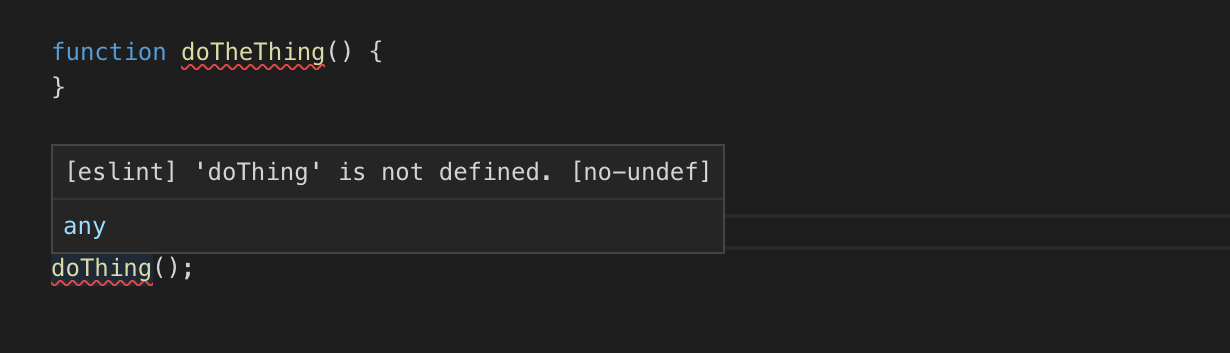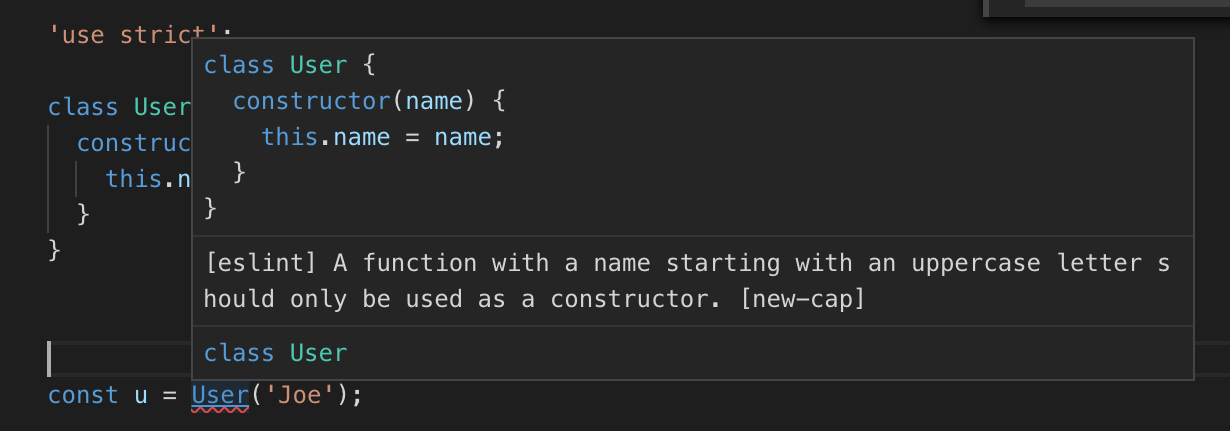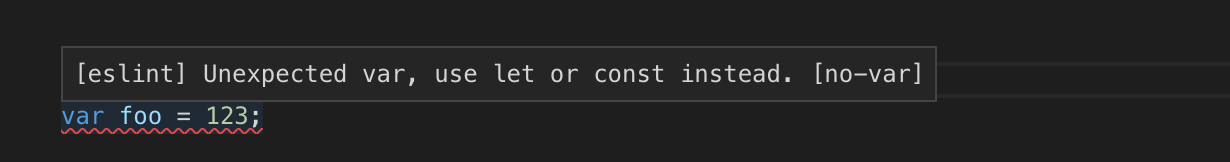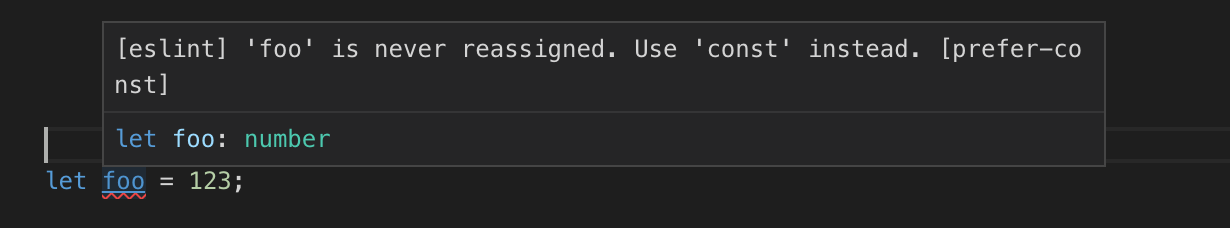# 先决条件

## You don't need `onbody`

```<body onload="somefunction()">
```

```<html>
...
<body>
...
</body>
<script>
// inline javascript
</script>
</html>
```

## 了解闭包如何工作

```function a(v) {
const foo = v;
return function() {
return foo;
};
}

const f = a(123);
const g = a(456);
console.log(f());  // prints 123
console.log(g());  // prints 456
```

## 理解`this`的工作原理

`this`并不是什么魔法。它实际上像是一个像被自动传给函数的参数一样的变量。 简单的说就是像这样直接调用函数

```somefunction(a, b, c);
```

`this`将会为`null` (使用严格模式时) 当你使用`.`操作符像这样调用函数时

```someobject.somefunction(a, b, c);
```

`this`将会被设置为`someobject`

``` const callback = someobject.somefunction;
```

``` const callback = someobject.somefunction.bind(someobject);
```

## ES5/ES6/ES7 特性

### 使用`for(elem of collection)`而不是`for(elem in collection)`

`for of`是新的，`for in`是旧的。 `for of`解决了`for in`的问题。

```for (const [key, value] of Object.entries(someObject)) {
console.log(key, value);
}
```

### 使用解构赋值

``` const width = dims.width;
const height = dims.height;
```

``` const {width, height} = dims;
```

### 使用对象声明简写

``` const width = 300;
const height = 150;
const obj = {
width: width,
height: height,
area: function() {
return this.width * this.height
},
};
```

``` const width = 300;
const height = 150;
const obj = {
width,
height,
area() {
return this.width * this.height;
},
};
```

### 使用扩展运算符`...`

``` function log(className, ...args) {
const elem = document.createElement('div');
elem.className = className;
elem.textContent = [...args].join(' ');
document.body.appendChild(elem);
}
```

```const position = [1, 2, 3];
somemesh.position.set(...position);
```

### 理解 getters 和 setters

Getterssetters是 在大多数现代语言中常见的。ES6`class`语法 让他们比ES6之前的更容易。

### 合理使用箭头函数

```loader.load((texture) => {
// use textrue
});
```

```(function(args) {/* code */}).bind(this))
```

### Promises 以及 async/await

Promises改善异步代码的处理。Async/await改善 promise的使用。

### 使用模板字符串

```const foo = `this is a template literal`;
```

```const foo = `this
is
a
template
literal`;
const bar = "this\nis\na\ntemplate\nliteral";
```

```const r = 192;
const g = 255;
const b = 64;
const rgbCSSColor = `rgb(\${r},\${g},\${b})`;
```

or

```const color = [192, 255, 64];
const rgbCSSColor = `rgb(\${color.join(',')})`;
```

or

```const aWidth = 10;
const bWidth = 20;
someElement.style.width = `\${aWidth + bWidth}px`;
```

# 考虑使用Visual Studio Code